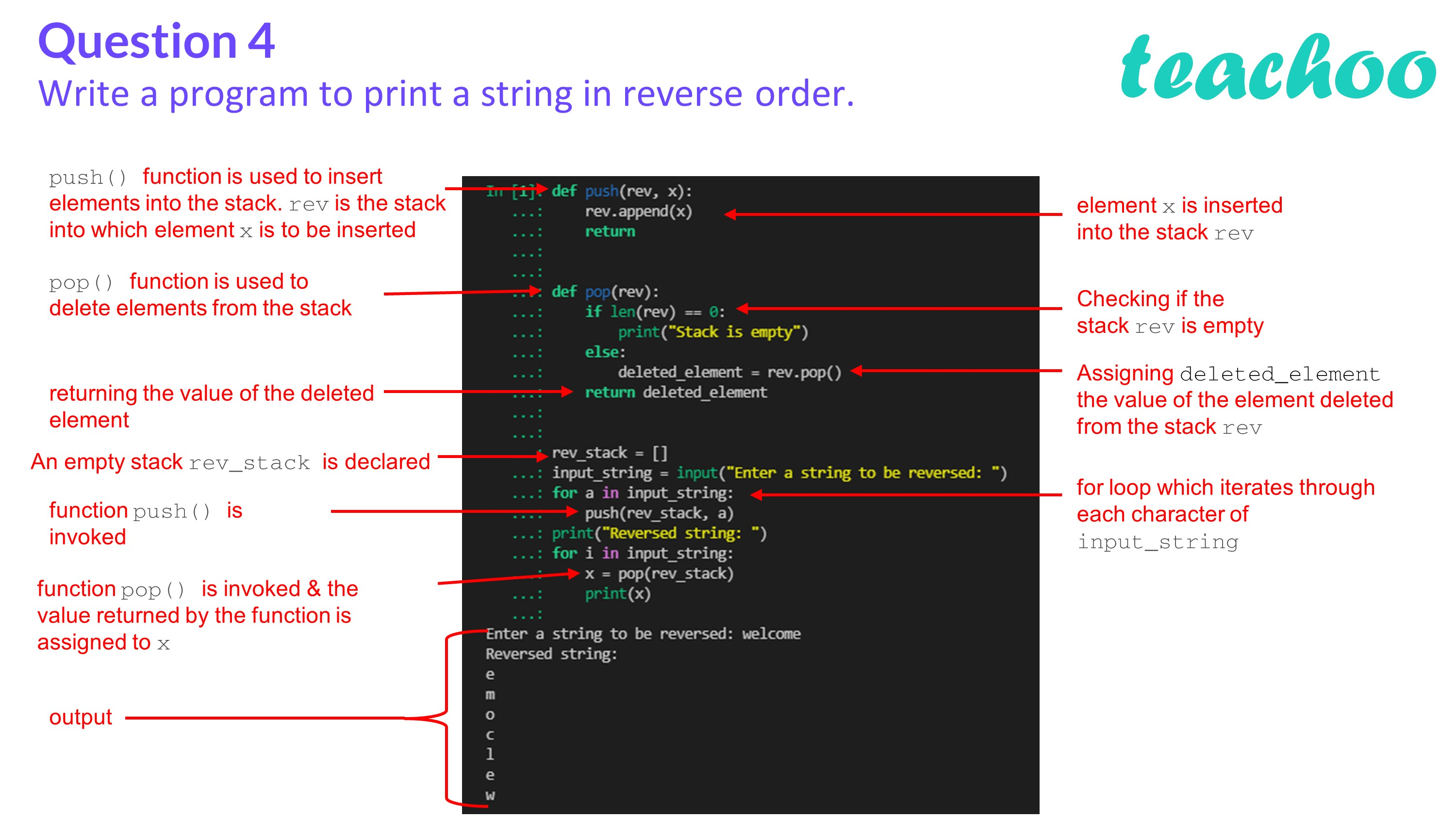Past Year - 2 Mark Questions

Computer Science - Class 12
Chapter 3 - Stacks

## Write a program to print a string in reverse order.

To print a string in reverse order, first insert each character of the string into a stack. Then, delete each element from the top of the stack and print the deleted element.

Code:

``` def push(rev,x): ```

``` rev.append(x) ```

``` return ```

``` def pop(rev): ```

``` if len(rev)==0: ```

``` print("Stack is empty") ```

``` else: ```

``` deleted_element=rev.pop() ```

``` return deleted_element ```

``` rev_stack=[] ```

``` input_string=input("Enter a string to be reversed: ") ```

``` for a in input_string: ```

``` push(rev_stack,a) ```

``` print("Reversed string: ") ```

``` for i in input_string: ```

``` x=pop(rev_stack) ```

``` print(x) ```

Output:

``` Enter a string to be reversed: welcome ```

``` Reversed string: ```

``` e ```

``` m ```

``` o ```

``` c ```

``` l ```

``` e ```

``` w ```Learn in your speed, with individual attention - Teachoo Maths 1-on-1 Class

### Transcript

Write a program to print a string in reverse order. Answer: To print a string in reverse order, first insert each character of the string into a stack. Then, delete each element from the top of the stack and print the deleted element. Code: def push(rev,x): rev.append(x) return def pop(rev): if len(rev)==0: print("Stack is empty") else: deleted_element=rev.pop() return deleted_element rev_stack=[] input_string=input("Enter a string to be reversed: ") for a in input_string: push(rev_stack,a) print("Reversed string: ") for i in input_string: x=pop(rev_stack) print(x) Output: Enter a string to be reversed: welcome Reversed string: e m o c l e w